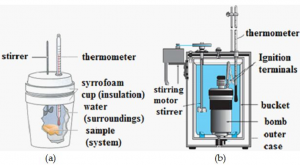Checkout JEE MAINS 2022 Question Paper Analysis : Checkout JEE MAINS 2022 Question Paper Analysis :

# Measurement Of Enthalpy And Internal Energy Change

Generally, measurement of enthalpy and internal energy is done by an experimental technique known as calorimetry. Calorimetry techniques are based on thermometric methods carried out in a vessel called calorimeter which is immersed in a known volume of liquid. The heat evolved in the process is generally calculated with the help of known heat capacities of the liquid and the calorimeter by measuring the temperature differences. Two different conditions under which these measurements are made are:

• At constant pressure, qp
• At constant volume, qv

## Measurement of enthalpy change

Enthalpy is defined as the energy released at constant pressure. Mathematically, it is the sum of internal energy with the product of pressure and volume.

H= U+ PV

Where,

H= enthalpy

U= internal energy

P= pressure of the system

V= volume of the system

It is quite impossible to calculate the absolute enthalpy of a system directly. Hence, we calculate the enthalpy about a reference point. Therefore, we generally measure the change in enthalpy. Enthalpy change, ΔH is positive for endothermic reactions while it is negative for exothermic reactions. Measurement of enthalpy change in the laboratory is done through calorimetry techniques. We know that enthalpy change is the heat change at constant pressure that isΔH= qp. “Coffee-cup calorimeter” is often used to calculate the enthalpy change. In this technique, the cup is partially filled with a known volume of water and a thermometer is inserted through the lid of the cup such that its bulb is below the water surface.When a chemical reaction occurs, the heat of reaction is absorbed by the water. The change in the water temperature is used to calculate the amount of heat that has been absorbed or evolved. Since the cup is made of polystyrene foam, a very good insulator, very little heat energy escapes. Energy change or enthalpy change in this process is calculated as:

ΔH=qp= mcpΔT

Where,

m= mass of water

cp= specific heat capacity of water at constant pressure

ΔT = temperature difference## Measurement of change in internal energy

Internal energy change is the energy change at constant volume. A bomb calorimeter is generally used for the measurement of internal energy change. In this technique, a steel vessel (commonly called bomb) is immersed in a water bath in order to ensure that no heat is lost to the surrounding. A combustible substance is burnt in oxygen gas supplied in the bomb. The heat evolved is absorbed by the water around the bomb and the change in temperature is measured. Energy changes associated with the reaction are measured at constant volume as volume doesn’t change in the completely sealed bomb calorimeter. As the volume remains constant, work done is zero for the system. Energy change or internal energy change in the process is calculated as:

ΔU=qv= mcvΔT

Where,

m= mass of water

cv= specific heat capacity at constant volume

ΔT = temperature differenceFor detailed discussions on the calculation of enthalpy and internal energy, register with BYJU’S.

Test your Knowledge on Measurement of enthalpy and internal energy change!